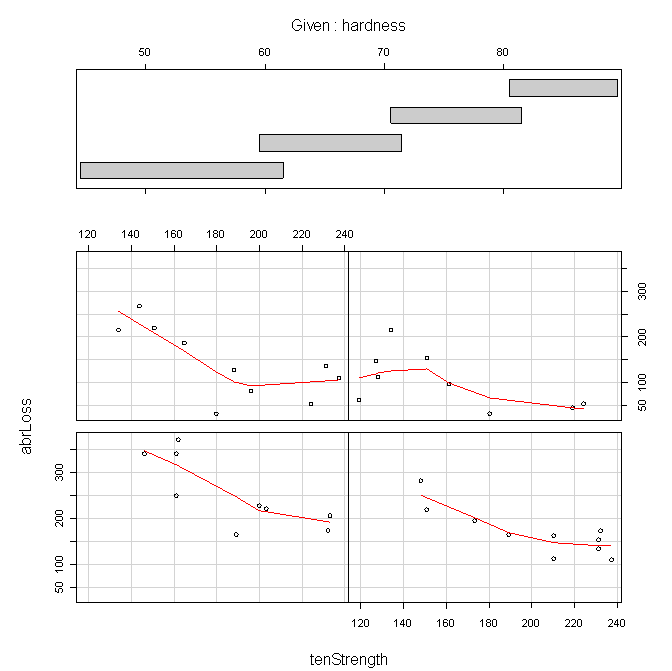Mike Livermore – CSI 773 – Week 11 Assignment – Regression Diagnostics – November 2010

3 – One D distribution view of variable Abrasion Loss4 – Two Dimension Scatterplots of 3 variables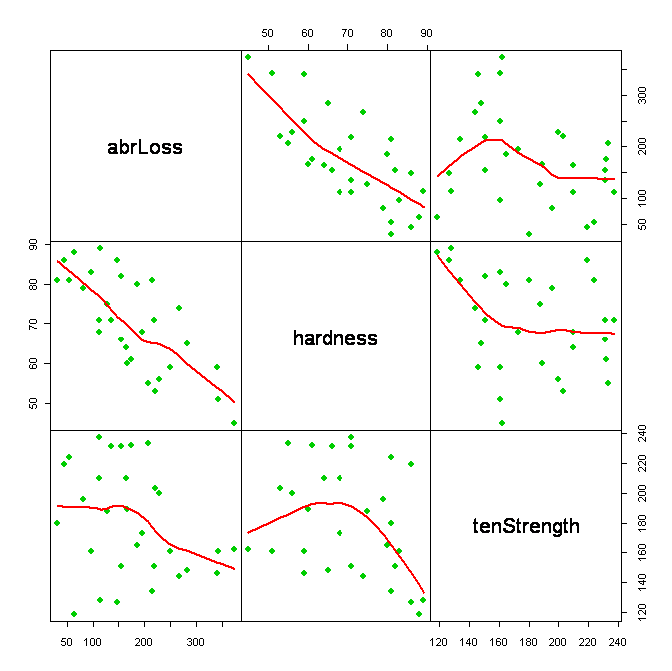5 – Plot of Linear regression example with two extreme outliers (high leverage points)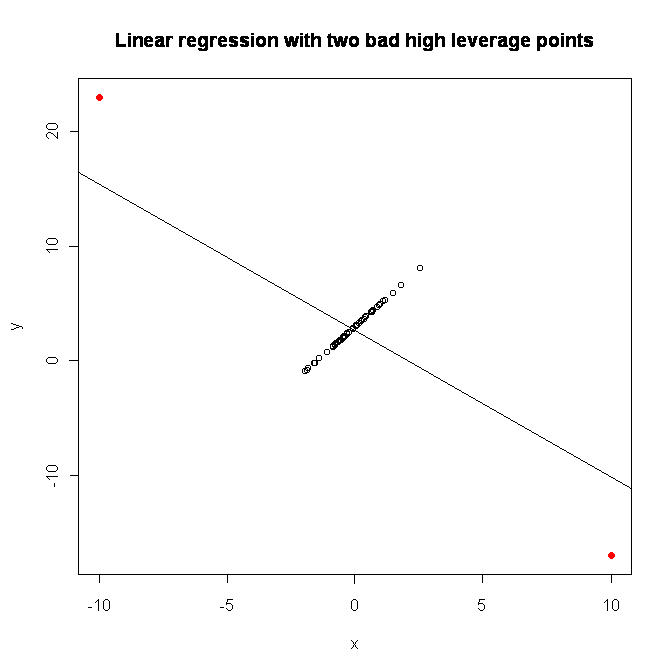7.2 – Abrasion Loss Model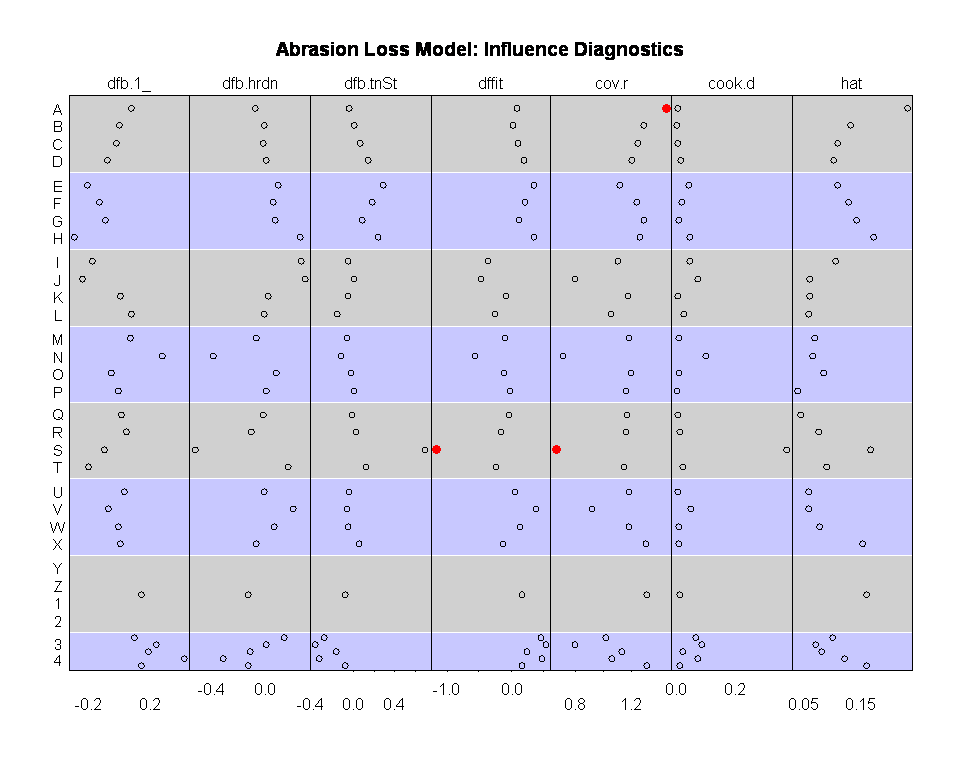7.3 – Unusual Residual Diagnostic Plot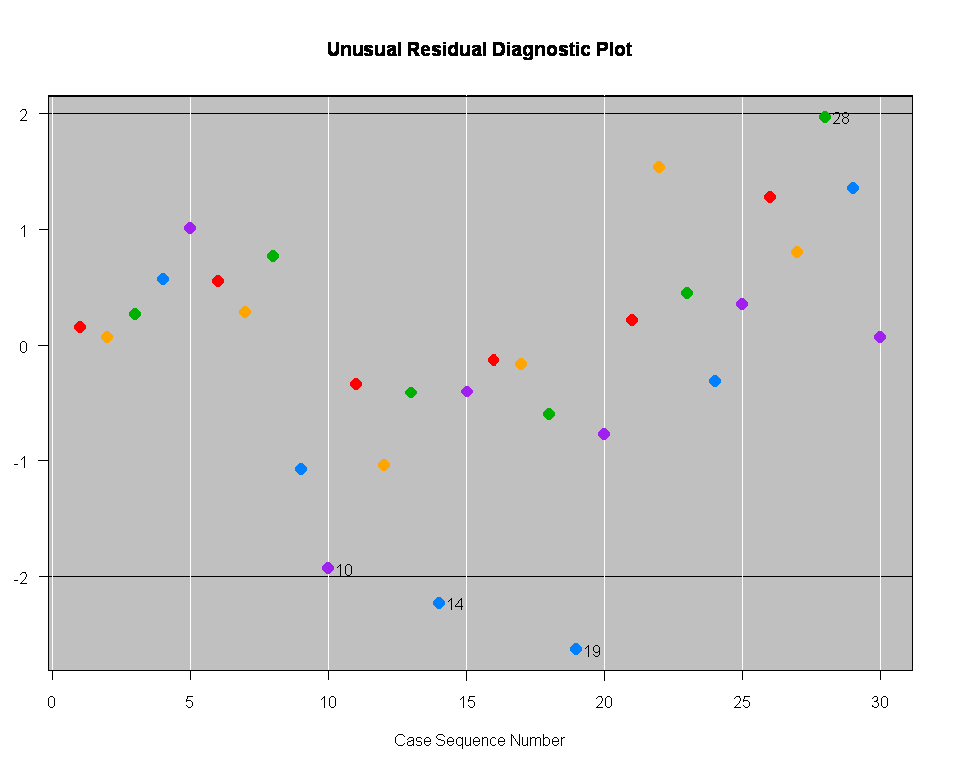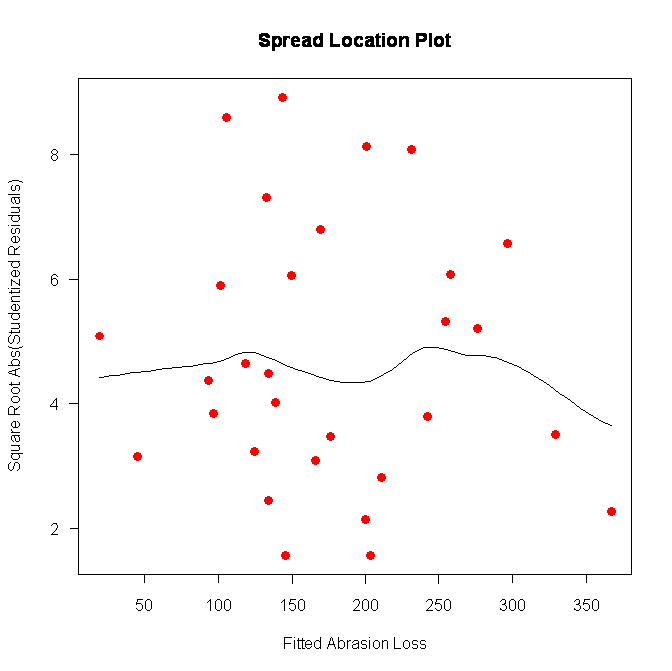7.5 – Residuals QQ Plot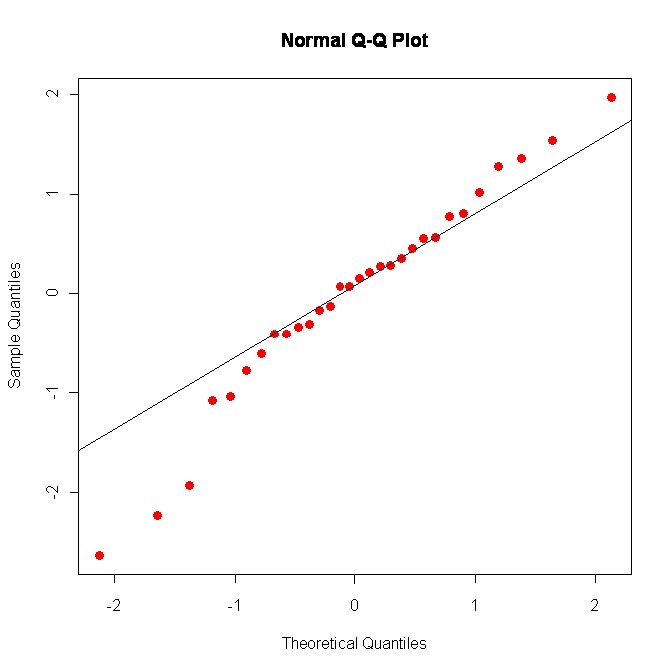7.6 – Partial residuals plot of Abrasion Loss and Adjusted Hardness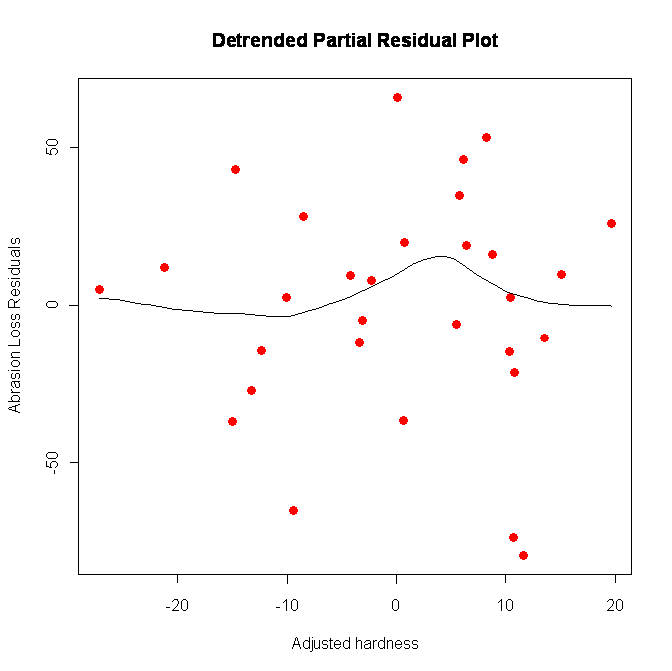8.3 – Original 3D plot and Rotated by x=-60 and z=55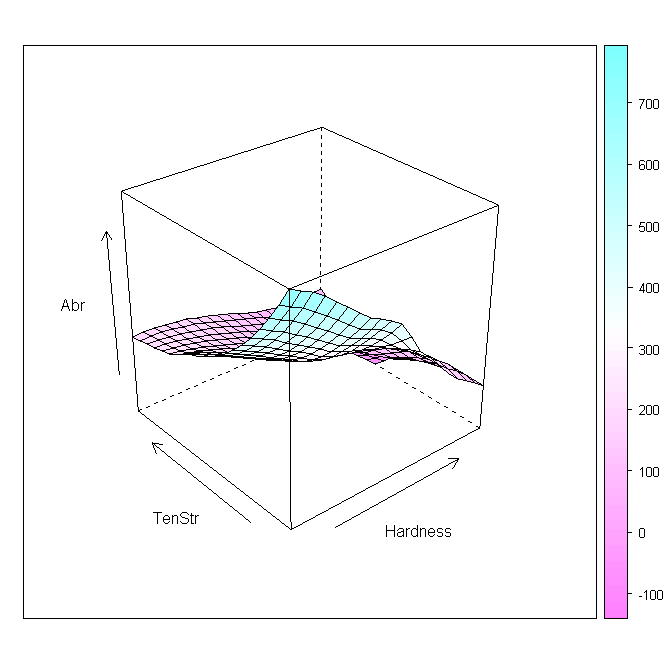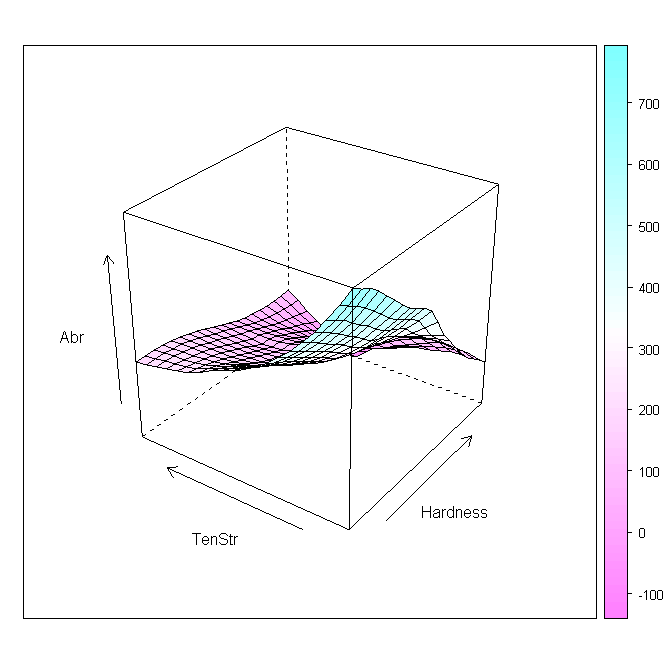8.3 – Contour plot9 – Abrasion Loss and Hardness conditioned by Tensile Strength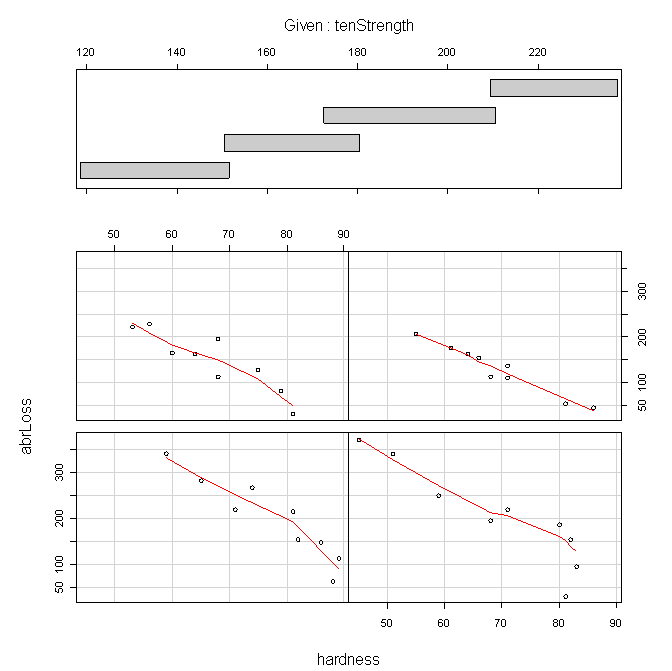9 – Abrasion Loss and Tensile Strength conditioned by Hardness